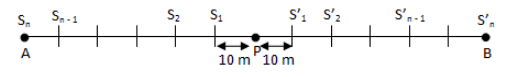# Along a road lie an odd number of stones placed at intervals of 10 metres

Along a road lie an odd number of stones placed at intervals of 10 metres. These stones have to be assembled around the middle stone. A person can carry only one stone at a time. A man carried the job with one of the end stones by carrying them in succession. In carrying all the stones he covered a distance of 3 km. Find the number of stones.

Let there be (2n + 1) stones.
Now, one stone lies in the middle and n stones on each side of ir in a row.
Let P be the middle stone and let A and B be the end stones as shown below:Clearly, there are n intervals each of length 10 m on both the sides of P.
Now, suppose the man starts from A. He picks up the end stone on the left of mid - scone
and goes to the mid-stone, drops it and goes to (n-1)the stone on left, picks it up, goes to
the mid-stone and drops it.
The eprocess is repeated till he collects all stones on the left of mid-stone or the mid-stone.
So, distance covered in collecting stones on the left of the mid—stones is
10×n+2[10×(n-1)+10×(n-2)+…+10×2+10×1]
After collecting all stones on the if t, he goes co che stone B and repeats the same process.
So, distance covered in collecting the stones on right side of the mid—stone
2[10×n+10×(n-1) + 10×(n-2)+ … + 10×2+10×1)
Toral distance covered
l0×n+2[10×(n-1) + 10×(n-2)+ … + 10×2+10×1] +2[10×n]+10×(n-1) + 10×(n-2)+ … + 10×2+10×1]
4[10×n+10×(n-1)+ …+10×2+10×1]-10×n
=40[1+2+3+ . +n]-10n
= 40[n/2-(1 +n)]-10n
20n(n+ 1)-10n
20n2+ 10n
Sur the toral distance covered is 3 km, i.e. 3000 m
20n2+lOn = 3000
2n2+n—300 0
(n -12)(2n +25)= O
n = 12
Thus, teh number of stones = 2n + 1 = 25